Activation Functions : Sigmoid, ReLU, Leaky ReLU and Softmax basics for Neural Networks and Deep…Let’s start with the basics of Neurons and Neural Network and What is an Activation Function and Why we would need it >

Neurons make up an Artificial Neural Network and a Neuron can be visualized as something that is holding a number which comes from the ending branches (Synapses) supplied at that Neuron, what happens is for a Layer of Neural Network we multiply the input to the Neuron with the weight held by that synapse and sum all of those up.

Example Code for Forward Propagation in a Single Neuron :

Neuron.py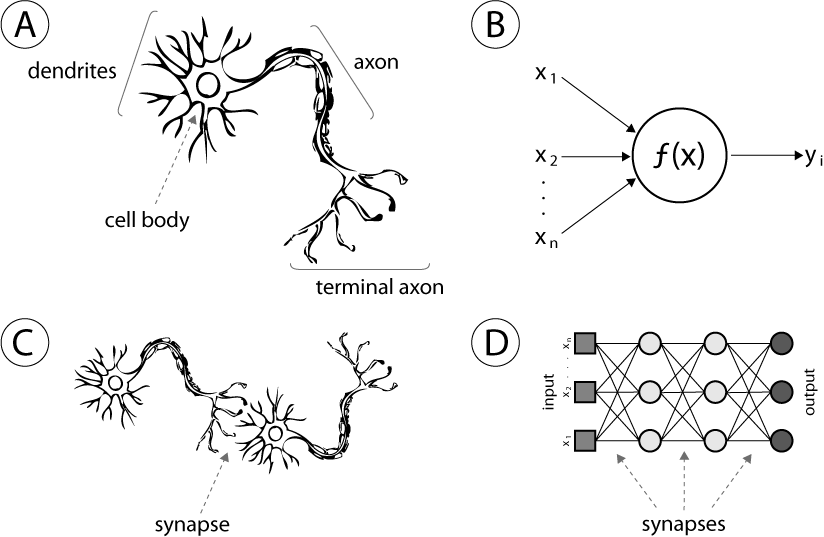Synapses and Neurons in Neural Networks both Biological and Computational

For example (see D in above figure), if the weights are w1, w2, w3 …. wN and inputs being i1, i2, i3 …. iN we get a summation of : w1*i1 + w2*i2 + w3*i3 …. wN*iN

For several layers of Neural Networks and Connections we can have varied values of wX and iX and the summation S which varies according to whether the particular Neuron is activated or not, so to normalize this and prevent drastically different range of values, we use what is called a Activation Function for Neural networks that turns these values into something equivalent between 0,1 or -1,1 to make the whole process statistically balanced.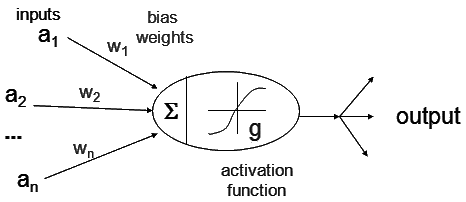Input, Weights and Output

Activation functions that are commonly used based on few desirable properties like :

• Nonlinear — When the activation function is non-linear, then a two-layer neural network can be proven to be a universal function approximator. The identity activation function does not satisfy this property. When multiple layers use the identity activation function, the entire network is equivalent to a single-layer model.
• Range — When the range of the activation function is finite, gradient-based training methods tend to be more stable, because pattern presentations significantly affect only limited weights. When the range is infinite, training is generally more efficient because pattern presentations significantly affect most of the weights. In the latter case, smaller learning rates are typically necessary.
• Continuously differentiable — This property is desirable (ReLU is not continuously differentiable and has some issues with gradient-based optimization, but it is still possible) for enabling gradient-based optimization methods. The binary step activation function is not differentiable at 0, and it differentiates to 0 for all other values, so gradient-based methods can make no progress with it.

Derivative or Differential or Slope: Change in y-axis according to change in x-axis.

• Monotonic — When the activation function is monotonic, the error surface associated with a single-layer model is guaranteed to be convex.

Monotonic function: A function which is either entirely non-increasing or non-decreasing.

• Smooth functions with a monotonic derivative — These have been shown to generalize better in some cases.
• Approximates identity near the origin — When activation functions have this property, the neural network will learn efficiently when its weights are initialized with small random values. When the activation function does not approximate identity near the origin, special care must be used when initializing the weights.

See table of Activation Functions >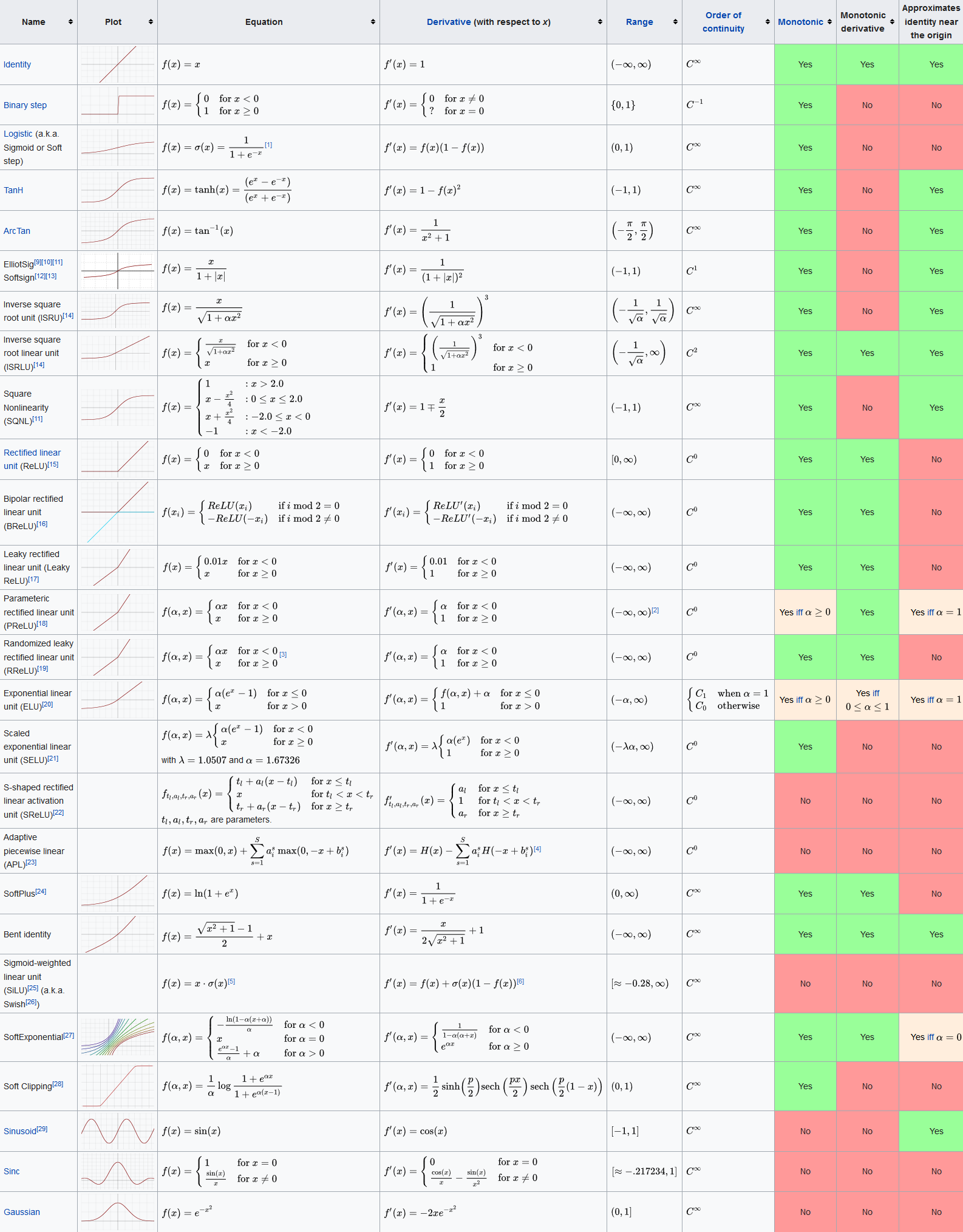Activation Function for Neural Networks

Breaking down some Activation functions :

1. The Sigmoid Function >

Sigmoid functions are used in machine learning for the logistic regression and basic neural network implementations and they are the introductory activation units. But for advanced Neural Network Sigmoid functions are not preferred due to various drawbacks.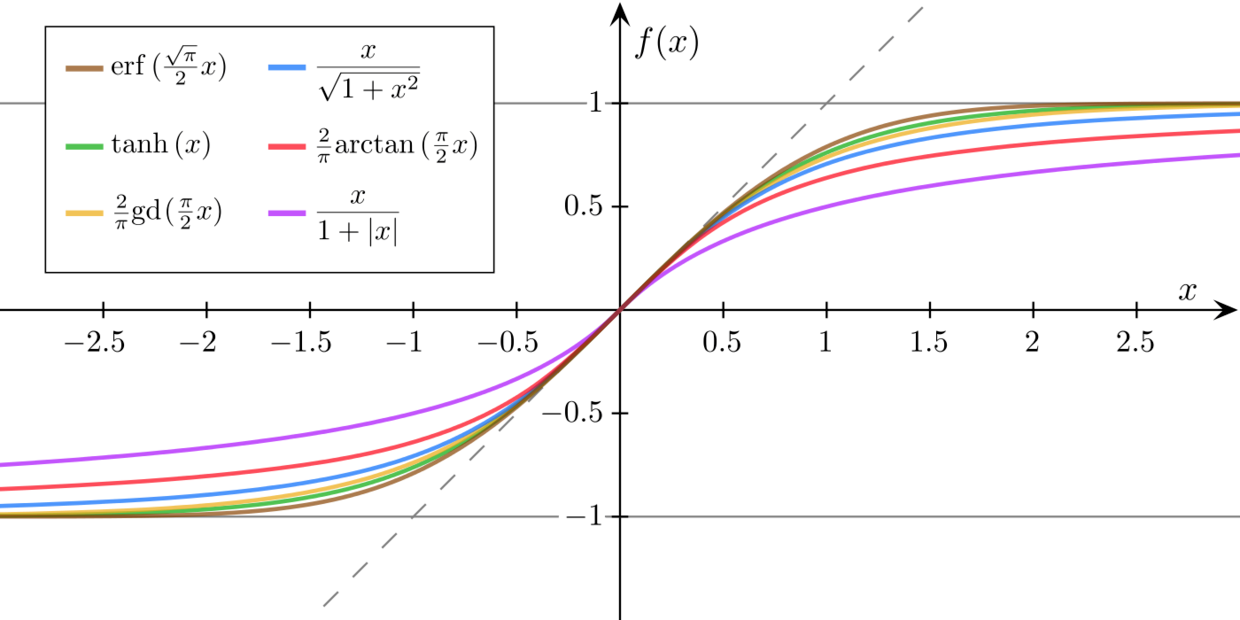Sigmoid Function

Although sigmoid function and it’s derivative is simple and helps in reducing time required for making models, there is a major drawback of info loss due to the derivative having a short range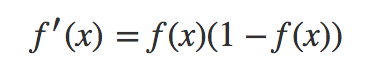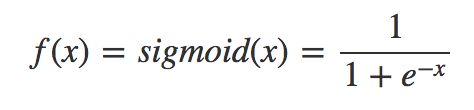Sigmoid and it’s derivative function

Therefore the more layers there are in our Neural Network or the deeper our Neural Network is, the more info is compressed and lost at each layer and this amplifies and causes major data loss overall.

Besides the logistic function, sigmoid functions include the ordinary arctangent, the hyperbolic tangent, the Gudermannian function, and the error function, but also the generalised logistic function and algebraic functions

2. Rectified Linear Units (ReLU) >

The rectifier is, as of 2018, the most popular activation function for deep neural networks.

Most Deep Learning applications right now make use of ReLU instead of Logistic Activation functions for Computer Vision, Speech Recognition and Deep Neural Networks etc.

Some of the ReLU variants include : Softplus (SmoothReLU), Noisy ReLU, Leaky ReLU, Parametric ReLU and ExponentialReLU (ELU).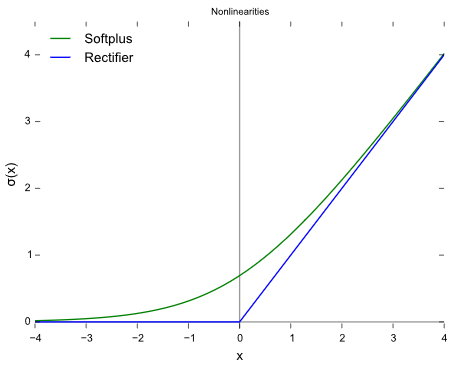ReLU

ReLU : A Rectified Linear Unit (A unit employing the rectifier is also called a rectified linear unit ReLU) has output 0 if the input is less than 0, and raw output otherwise. That is, if the input is greater than 0, the output is equal to the input. The operation of ReLU is closer to the way our biological neurons work.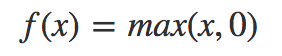ReLU f(x)

ReLU is non-linear and has the advantage of not having any backpropagation errors unlike the sigmoid function, also for larger Neural Networks, the speed of building models based off on ReLU is very fast opposed to using Sigmoids :

• Biological plausibility: One-sided, compared to the antisymmetry of tanh.
• Sparse activation: For example, in a randomly initialized network, only about 50% of hidden units are activated (having a non-zero output).
• Better gradient propagation: Fewer vanishing gradient problems compared to sigmoidal activation functions that saturate in both directions.
• Efficient computation: Only comparison, addition and multiplication.
• Scale-invariant: max ( 0, a x ) = a max ( 0 , x ) for a ≥ 0

ReLUs aren’t without any drawbacks some of them are that ReLU is Non Zero centered and is non differentiable at Zero, but differentiable anywhere else.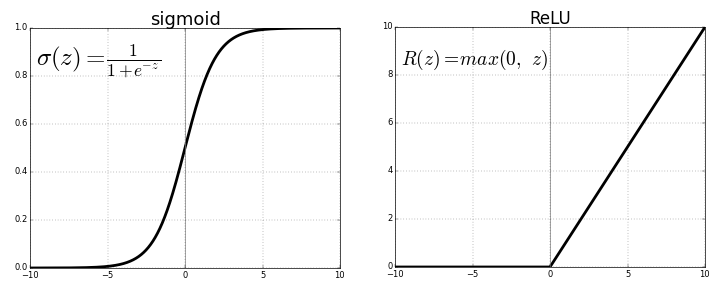Sigmoid Vs ReLU

Another problem we see in ReLU is the Dying ReLU problem where some ReLU Neurons essentially die for all inputs and remain inactive no matter what input is supplied, here no gradient flows and if large number of dead neurons are there in a Neural Network it’s performance is affected, this can be corrected by making use of what is called Leaky ReLU where slope is changed left of x=0 in above figure and thus causing a leak and extending the range of ReLU.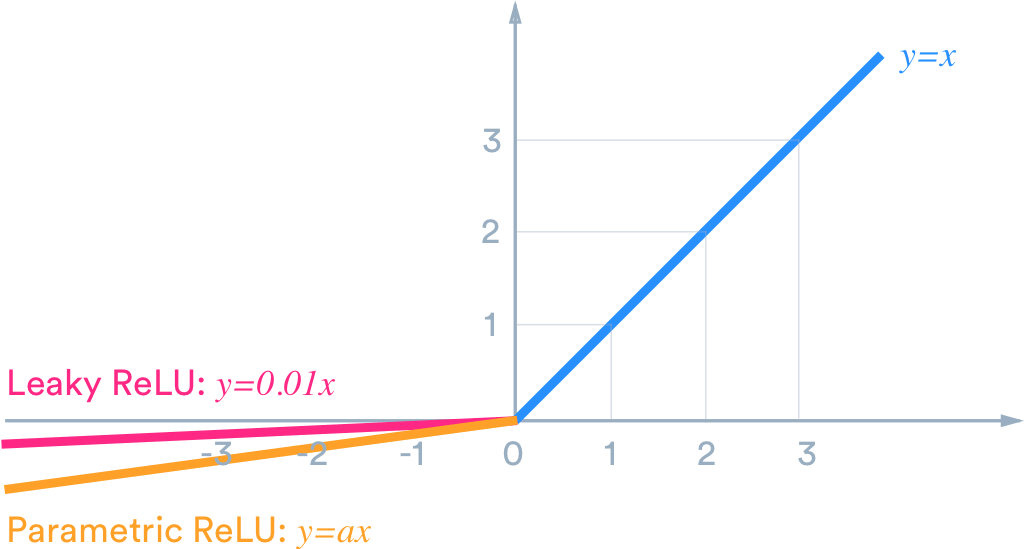Leaky ReLU

3. Softmax >

Softmax is a very interesting activation function because it not only maps our output to a [0,1] range but also maps each output in such a way that the total sum is 1. The output of Softmax is therefore a probability distribution.

From Wikipedia >

The softmax function is often used in the final layer of a neural network-based classifier. Such networks are commonly trained under a log loss (or cross-entropy) regime, giving a non-linear variant of multinomial logistic regression.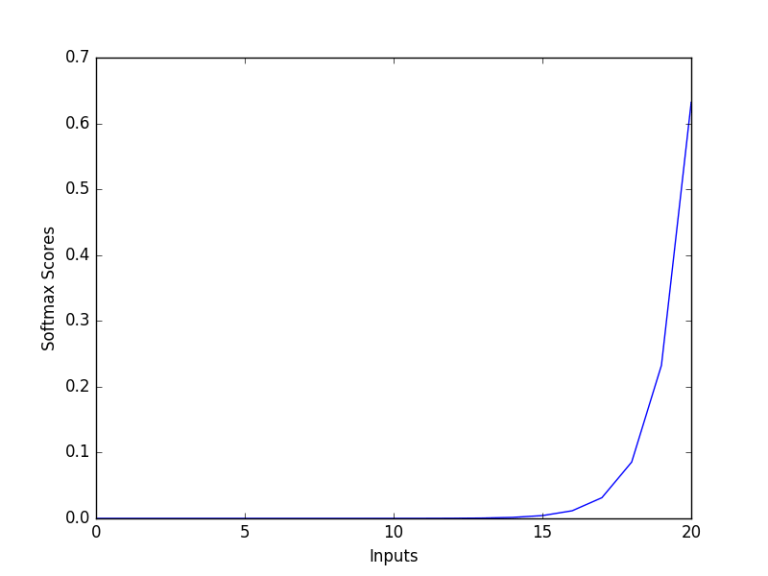Softmax Graphed

Mathematically Softmax is the following function where z is vector of inputs to output layer and j indexes the the output units from 1,2, 3 …. k :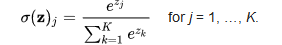Softmax Function

In conclusion, Softmax is used for multi-classification in logistic regression model whereas Sigmoid is used for binary classification in logistic regression model, the sum of probabilities is One for Softmax. Also, i.

For deeper understanding of all the main Activation Functions I would advise you to graph them in Python/MATLAB/R their derivatives too and think of their Ranges and Minimum and Maximum Values, and how these are affected when numbers are multiplied with them.